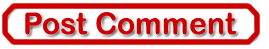Best of the Week# Fibonacci in Nature: The Golden Ratio and the Golden Spiral

InvestorEducation / Learn to Trade Oct 11, 2012 - 12:30 PM GMT

By: EWIIf you've studied the financial markets, even for a short time, you've probably heard the term "Fibonacci numbers." The ratios and relationships derived from this mathematical sequence are applied to the markets to help determine targets and retracement levels.

Did you know that Fibonacci numbers are found in nature as well? In fact, we can see examples of the Fibonacci sequence all around us, from the ebb and flow of ocean tides to the shape of a seashell. Even our human bodies are examples of Fibonacci. Read more about the fascinating phenomenon of Fibonacci in nature.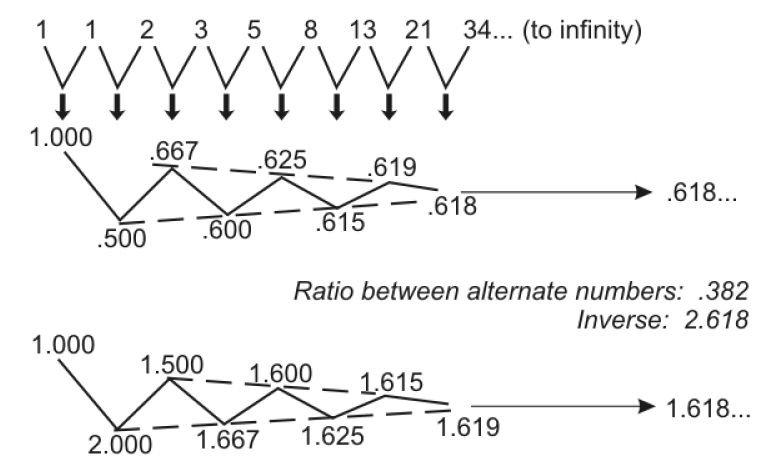Let's start with a refresher on Fibonacci numbers. If we start at 0 and then go to the next whole integer number, which is 1, and add 0 to 1, that gives us the second 1. If we then take that number 1 and add it again to the previous number, which is of course 1, we have 1 plus 1 equals 2. If we add 2 to its previous number of 1, then 1 plus 2 gives us 3, and so on. 2 plus 3 gives us 5, and we can do this all the way to infinity. This series of numbers, and the way we arrive at these numbers, is called the Fibonacci sequence. We refer to a series of numbers derived this way as Fibonacci numbers.

We can go back to the beginning and divide one number by its adjacent number - so 1�1 is 1.0, 1�2 is .5, 2�3 is .667, and so on. If we keep doing that all the way to infinity, that ratio approaches the number .618. This is called the Golden Ratio, represented by the Greek letter phi (pronounced "fie"). It is an irrational number, which means that it cannot be represented by a fraction of whole integers. The inverse of .618 is 1.618. So, in other words, if we carry the series forward and take the inverse of each of these numbers, that ratio also approaches 1.618. The Golden Ratio, .618, is the only number that will also be equal to its inverse when added to 1. So, in other words, 1 plus .618 is 1.618, and the inverse of .618 is also 1.618.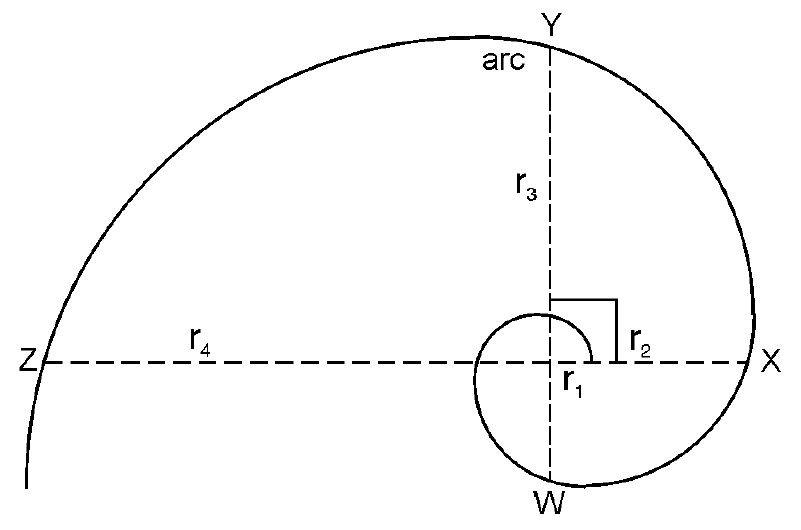This is a diagram of the Golden Spiral. The Golden Spiral is a type of logarithmic spiral that is made up of a number of Fibonacci relationships, or more specifically, a number of Golden Ratios. For example, if we take a specific arc and divide it by its diameter, that will also give us the Golden Ratio 1.618. We can take, for example, arc WY and divide it by its diameter of WY. That produces the multiple 1.618. Certain arcs are also related by the ratio of 1.618. If we take the arc XY and divide that by arc WX, we get 1.618. If we take radius 1 (r1), compare it with the next radius of an arc that's at a 90° angle with r1, which is r2, and divide r2 by r1, we also get 1.618.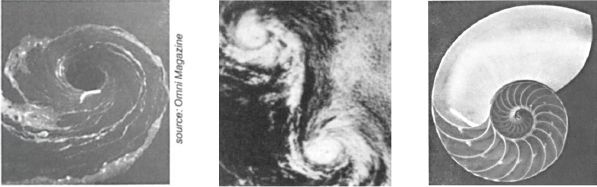Now here are some pictures of this Golden Spiral in various aspects of nature. For example, on the left is a whirlpool that displays the Golden Spiral and, therefore, these Fibonacci mathematical properties. We also see the Golden Spiral in the formation of hurricanes (center) and in the chambered nautilus shell (right), which also happens to be a common background that Elliott Wave International uses for a number of its presentations and graphics.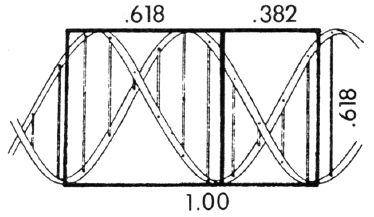We can also see the Golden Ratio in the DNA molecule. Research has shown that if you look at the height of the DNA molecule relative to its length, it is in the proportion of .618:1. If we look at the components of the DNA molecule, there is a major groove in the left section and a minor groove in the right section. The major groove is equal to .618 of the entire length of the DNA molecule, and the minor groove is equal to .382 of the entire length.

This graphic of the human body also shows how the Golden Ratio exists in certain relationships of the human anatomy.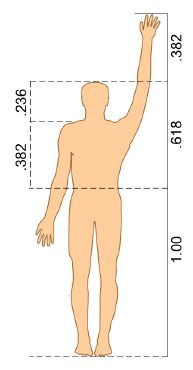Learn How You Can Use Fibonacci to Improve Your Trading If you'd like to learn more about Fibonacci and how to apply it to your trading strategy, download the entire 14-page free eBook, How You Can Use Fibonacci to Improve Your Trading. EWI Senior Tutorial Instructor Wayne Gorman explains: The Golden Spiral, the Golden Ratio, and the Golden Section How to use Fibonacci Ratios/Multiples in forecasting How to identify market targets and turning points in the markets you trade And more! See how easy it is to use Fibonacci in your trading. Download your free eBook today >>

Best Regards,

About the Publisher, Elliott Wave International
Founded in 1979 by Robert R. Prechter Jr., Elliott Wave International (EWI) is the world's largest market forecasting firm. Its staff of full-time analysts provides 24-hour-a-day market analysis to institutional and private investors around the world.

© 2005-2019 http://www.MarketOracle.co.uk - The Market Oracle is a FREE Daily Financial Markets Analysis & Forecasting online publication.